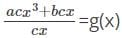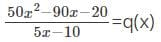ACT  >  Test: Polynomial Division

# Test: Polynomial Division

Test Description

## 10 Questions MCQ Test | Test: Polynomial Division

Test: Polynomial Division for ACT 2023 is part of ACT preparation. The Test: Polynomial Division questions and answers have been prepared according to the ACT exam syllabus.The Test: Polynomial Division MCQs are made for ACT 2023 Exam. Find important definitions, questions, notes, meanings, examples, exercises, MCQs and online tests for Test: Polynomial Division below.
Solutions of Test: Polynomial Division questions in English are available as part of our course for ACT & Test: Polynomial Division solutions in Hindi for ACT course. Download more important topics, notes, lectures and mock test series for ACT Exam by signing up for free. Attempt Test: Polynomial Division | 10 questions in 15 minutes | Mock test for ACT preparation | Free important questions MCQ to study for ACT Exam | Download free PDF with solutions
 1 Crore+ students have signed up on EduRev. Have you?
Test: Polynomial Division - Question 1

### If the polynomial f(x) = x2 + kx - 15, is exactly divisible by x - 5, then the value of k is _______

Detailed Solution for Test: Polynomial Division - Question 1

x+ kx - 15 is exactly divisible by x - 5
Dividing, x+ kx + 15 by x - 5
We get, 5k + 10 as remainder.
Since, x+ kx - 15 is exactly divisible by 2x - 5
∴ 5k + 10 = 0
k = -2

Test: Polynomial Division - Question 2

### The real number that should be subtracted from the polynomial f(x) = 15x5 + 70x4 + 35x3 - 135x2 - 40x - 11 so that it is exactly divisible by 5x4 + 10x3 - 15x2 - 5x is ____________

Detailed Solution for Test: Polynomial Division - Question 2

On dividing, 15x+ 70x+ 35x- 135x- 40x - 11 by 5x+ 10x- 15x- 5x
We get, 3x + 8 as quotient and remainder as -11.
So if we subtract -11 from 15x+ 70x+ 35x- 135x- 40x - 11 it will be exactly divisible by 5x+ 10x- 15x- 5x.

Test: Polynomial Division - Question 3

### The polynomial (x), if the divisor is 5x2, quotient is 2x + 3, and remainder is 10x + 20 is __________

Detailed Solution for Test: Polynomial Division - Question 3

We know that,
f(x) = q(x) × g(x) + r(x)
Where, f(x) is the dividend, q(x) is the quotient, g(x) is the divisor and r(x) is the remainder.
f(x) = 5x× (2x + 3) + 10x + 20
f(x) = 10x+ 15x+ 10x + 20

Test: Polynomial Division - Question 4

What will be the value of a and b if the polynomial f(x) = 30x- 50x+ 109x- 23x + 25, when divided by 3x- 5x + 10, gives 10x+ 3 as quotient and ax + b as remainder?

Detailed Solution for Test: Polynomial Division - Question 4

We know that,
f(x) = q(x) × g(x) + r(x)
Where, f(x) is the dividend, q(x) is the quotient, g(x) is the divisor and r(x) is the remainder.
∴ 30x- 50x+ 109x- 23x + 25 = (10x+ 3)(3x- 5x + 10) + ax + b
30x- 50x+ 109x- 23x + 25 = 30x- 50x+ 109x- 15x + 30 + ax + b
30x- 50x+ 109x- 23x + 25 - (30x- 50x+ 109x- 15x + 30) = ax + b
-23x + 25 + 15x - 30 = ax + b
-8x - 5 = ax + b
∴ a = -8, b = -5

Test: Polynomial Division - Question 5

If α is a zero of the polynomial f(x), then the divisor of f(x) will be _________

Detailed Solution for Test: Polynomial Division - Question 5

If α is a zero of the polynomial f(x).
The divisor will be x - α.
For example, if 5 is a zero of a polynomial f(x), then its divisor will be x - 5.

Test: Polynomial Division - Question 6

What real number that should be added to the polynomial f(x) = 81x- 31, so that it is exactly divisible by 9x + 1?

Detailed Solution for Test: Polynomial Division - Question 6

81x- 31 is exactly divisible by 9x + 1
Hence, on dividing 81x- 31 by 9x + 1
We get, 9x - 1 as quotient and remainder as -30.
So if we add 30 to 81x- 31, it will be exactly divisible by 9x + 1.

Test: Polynomial Division - Question 7

When a polynomial f(x) = acx+ bcx + d, is divided by g(x), it leaves quotient as cx, and remainder as d. The value of g(x)will be _____

Detailed Solution for Test: Polynomial Division - Question 7

We know that,
f(x) = q(x) × g(x) + r(x)
Where, f(x) is the dividend, q(x) is the quotient, g(x) is the divisor and r(x) is the remainder.
acx3 + bcx + d = cx × g(x) + d
acx3 + bcx + d – d = cx × g(x)g(x) = ax+ b

Test: Polynomial Division - Question 8

The quotient if the polynomial f(x) = 50x- 90x - 25 leaves a remainder of -5, when divided by 5x - 10, will be __________

Detailed Solution for Test: Polynomial Division - Question 8

We know that,
f(x) = q(x) × g(x) + r(x)
Where, f(x) is the dividend, q(x) is the quotient, g(x) is the divisor and r(x) is the remainder.
∴ 50x- 90x - 25 = q(x) × 5x - 10 - 5
50x- 90x - 25 + 5 = q(x) × 5x - 10We get, q(x) = 10x + 2

Test: Polynomial Division - Question 9

If two of the zeros of the polynomial f(x) = x+ (6 - √3)x+ (-1 - √3)x + 30 - 6√3 are 3 and -2 then, the other zero will be ____________

Detailed Solution for Test: Polynomial Division - Question 9

Since the zeros of the polynomial are 3 and -2.
The divisor of the polynomial will be (x - 3) and (x + 2).
Multiplying (x - 3) and (x + 2) = x+ 2x - 3x - 6 = x- x + 6
Dividing, x+ (6 - √3)x+ (-1 - √3)x + 30 - 6√3 by x- x + 6
We get, x - 5 + √3 as quotient.
Hence, the third zero will be 5 - √3.

Test: Polynomial Division - Question 10

If f(x) is divided by g(x), it gives quotient as q(x) and remainder as r(x). Then, f(x) = q(x) × g(x) + r(x) where, f(x) is the dividend, q(x) is the quotient, g(x) is the divisor and r(x) is the remainder.

Detailed Solution for Test: Polynomial Division - Question 10

Consider, f(x) is 27x- 39x, q(x) as 9x + 2, g(x) as 3x - 5 and remainder is 10.
f(x) = q(x) × g(x) + r(x)
RHS
q(x) × g(x) + r(x) = (9x + 2)(3x - 5) + 10 = 27x- 45x + 6x - 10 + 10 = 27x- 39x, which is equal to LHS.
Hence proved.

Information about Test: Polynomial Division Page
In this test you can find the Exam questions for Test: Polynomial Division solved & explained in the simplest way possible. Besides giving Questions and answers for Test: Polynomial Division, EduRev gives you an ample number of Online tests for practice(Scan QR code)